# Intermediate Jacobian

Any of a collection of complex tori determined by the odd-dimensional cohomology spaces of a complex Kähler manifold, and whose geometry is strongly related to the geometry of this manifold.

Let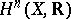(respectively,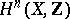) be the-dimensional cohomology space with real (respectively, integer) coefficients of a complex-analytic Kähler manifold. One can introduce a complex structure on the real torus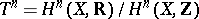ifis odd in two ways, using the representation of the-dimensional cohomology space with complex coefficients as a direct sum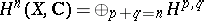of the spaces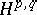of harmonic forms of type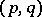. Let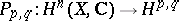be the projections, and letbe operators mapping the cohomology space with real coefficients into itself. Putting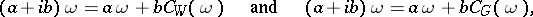for any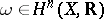,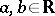, one obtains two complex structures on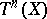. The first one,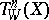, is called the Weil intermediate Jacobian, and the second,, is called the Griffiths intermediate torus. Ifis a Hodge variety, then the Hodge metric ofcanonically determines onthe structure of a polarized Abelian variety (cf. also Polarized algebraic variety; Abelian variety), which is not always true for. On the other hand, holomorphic variation of the manifoldimplies holomorphic variation of the intermediate tori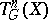, while Weil intermediate Jacobians need not have this property. The cup-product, giving a pairing between the spacesand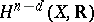with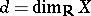, defines a complex pairing of the toriand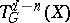, as well as a duality between the Abelian varietiesand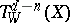. If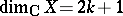, then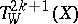is a self-dual Abelian variety with principal polarization, andis a principal torus.

The intermediate Jacobian is an important invariant of a Kähler manifold. If for two manifoldsandit follows from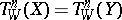(or from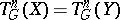) that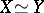, then one says that Torelli's theorem holds forand. Torelli's theorem holds, e.g., for algebraic curves. The irrationality of cubics in the projective space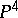(cf. ), as well as that of certain Fano varieties (cf. Fano variety), have been proved by means of the intermediate Jacobian.